Info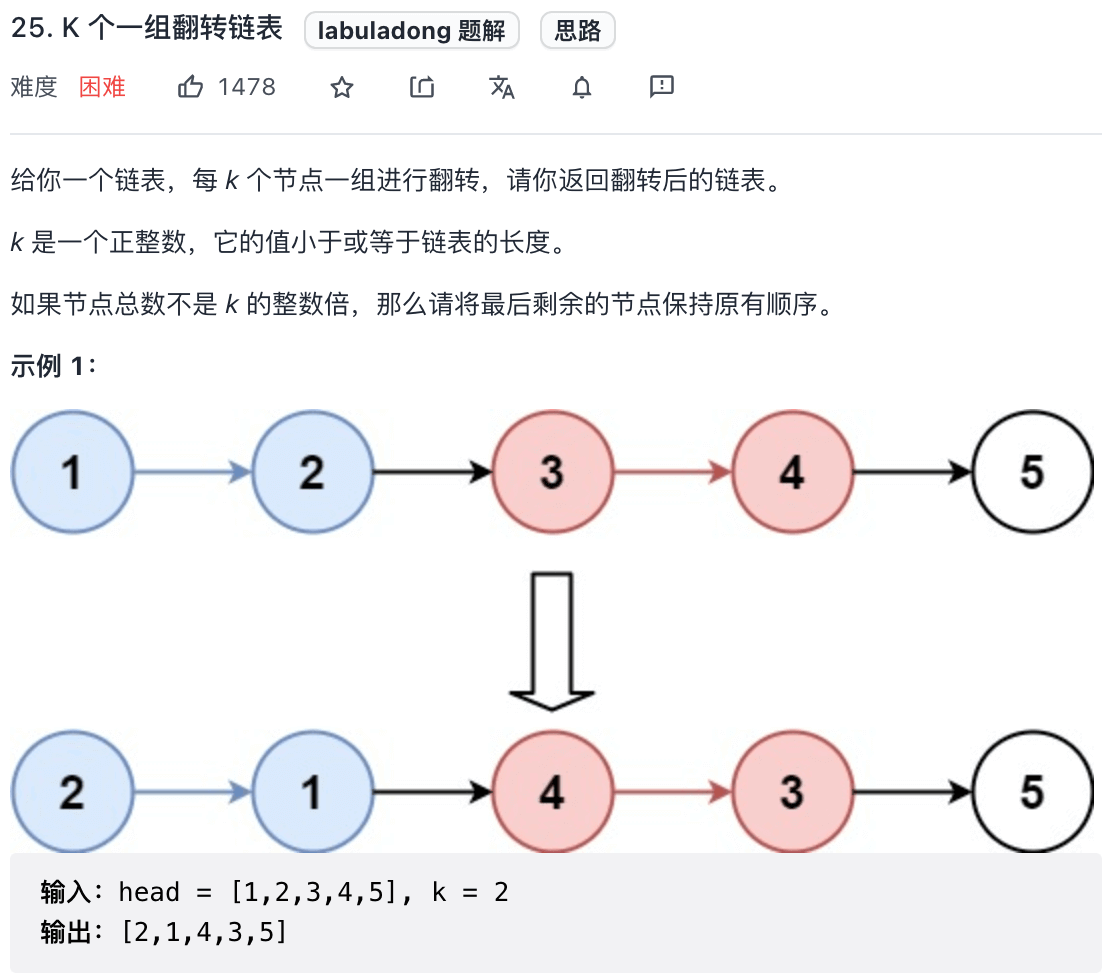### # 一、分析问题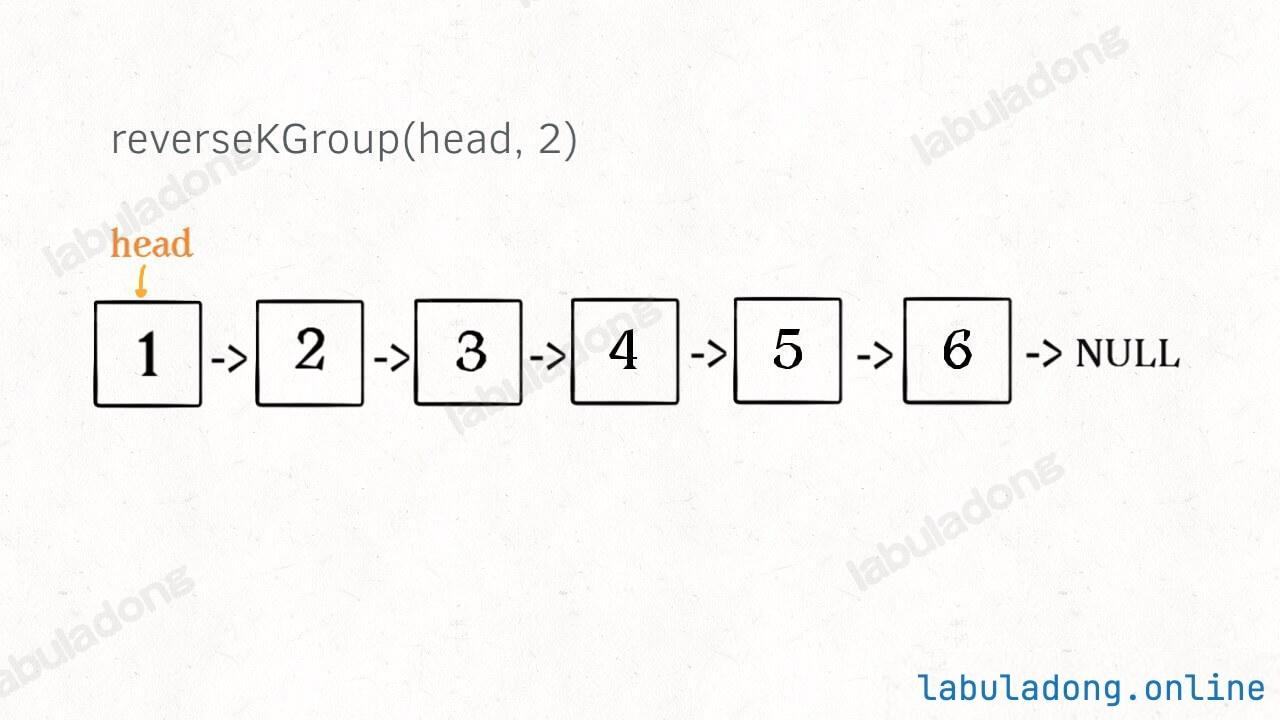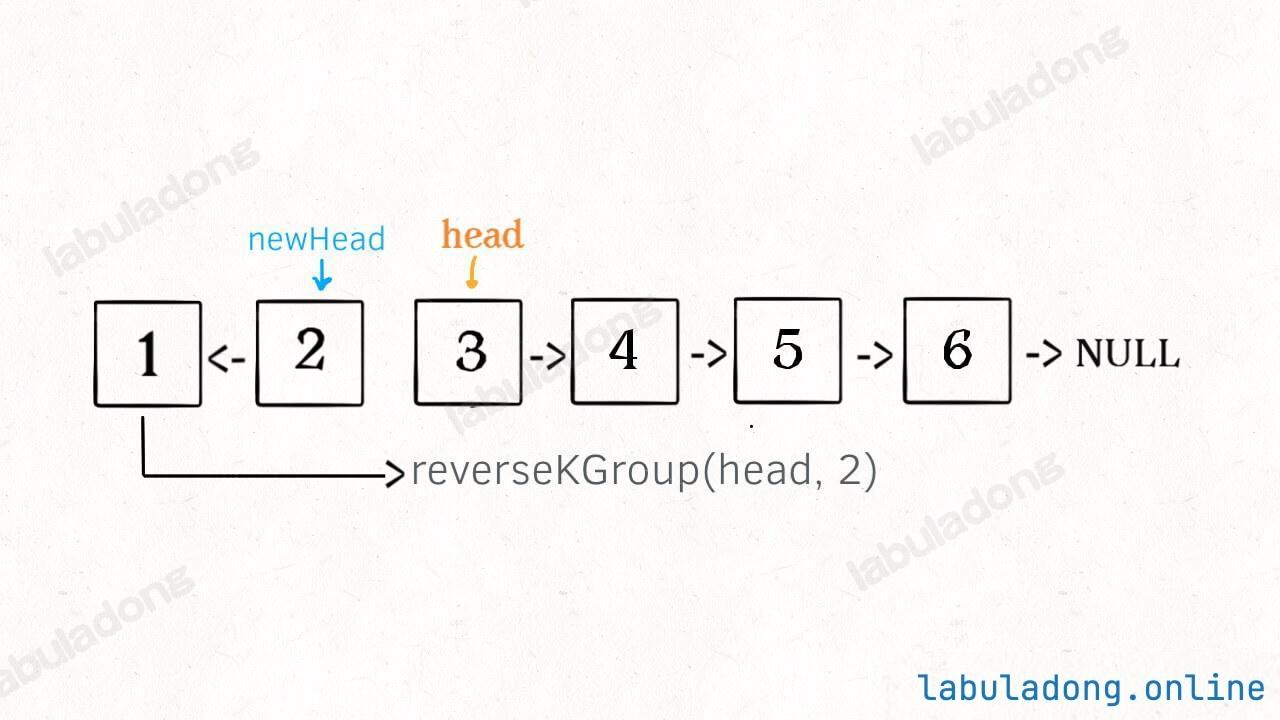1、先反转以 `head` 开头的 `k` 个元素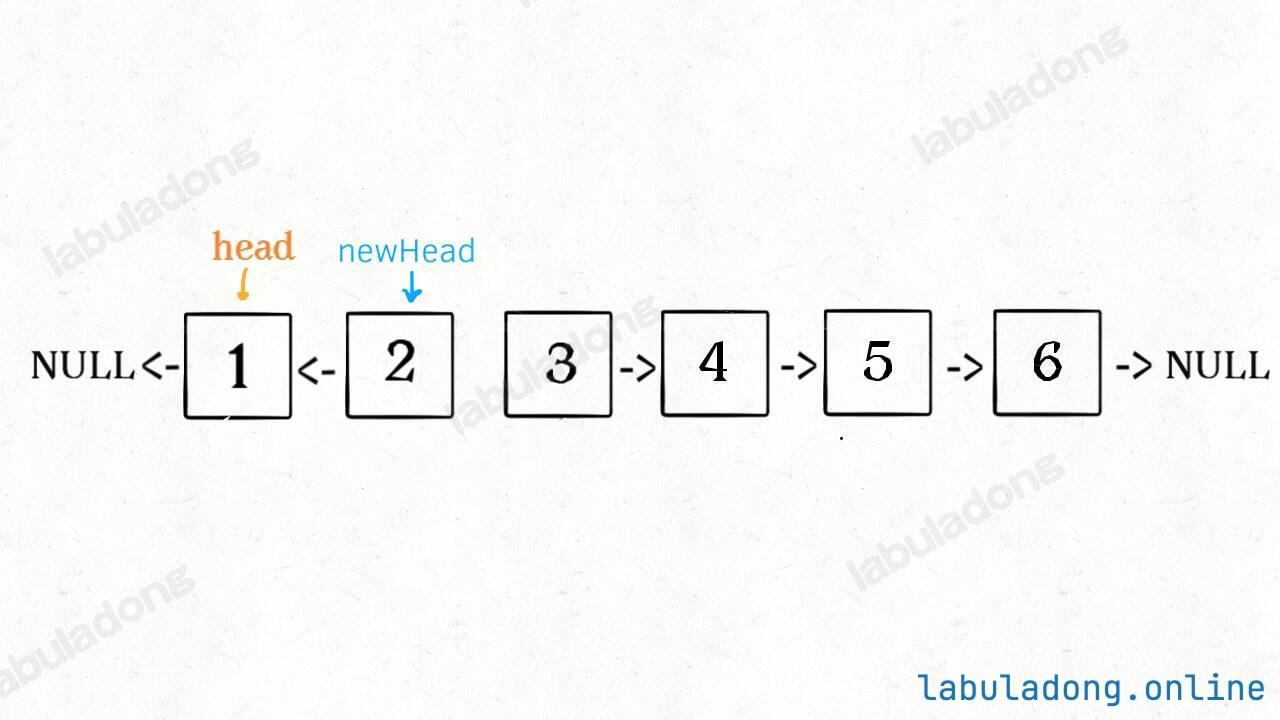2、将第 `k + 1` 个元素作为 `head` 递归调用 `reverseKGroup` 函数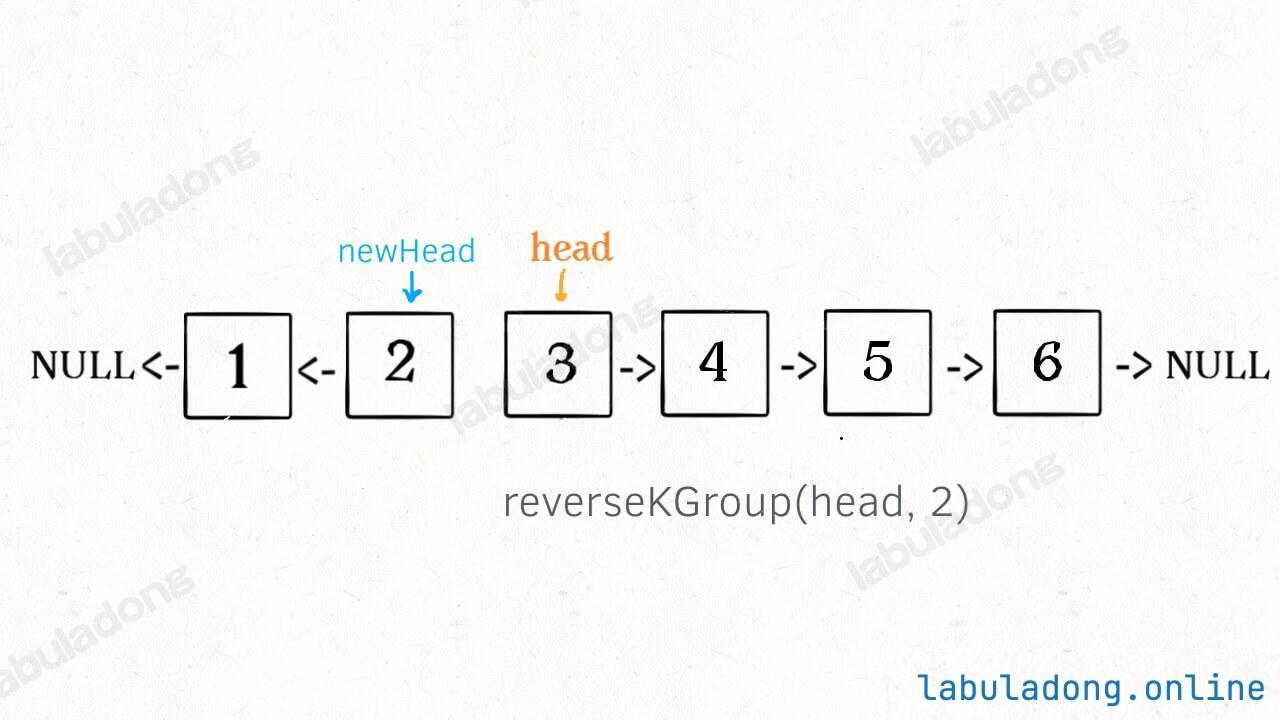3、将上述两个过程的结果连接起来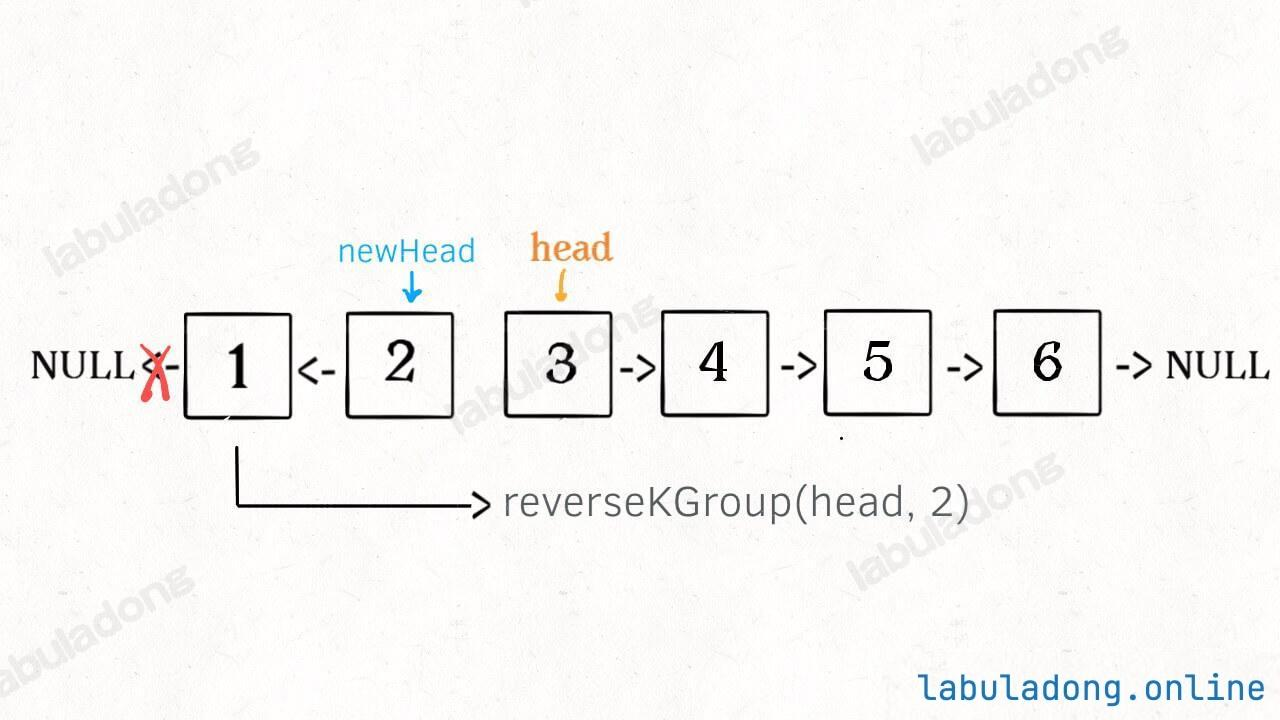### # 二、代码实现

``````// 反转以 a 为头结点的链表
ListNode reverse(ListNode a) {
ListNode pre, cur, nxt;
pre = null; cur = a; nxt = a;
while (cur != null) {
nxt = cur.next;
// 逐个结点反转
cur.next = pre;
// 更新指针位置
pre = cur;
cur = nxt;
}
// 返回反转后的头结点
return pre;
}
``````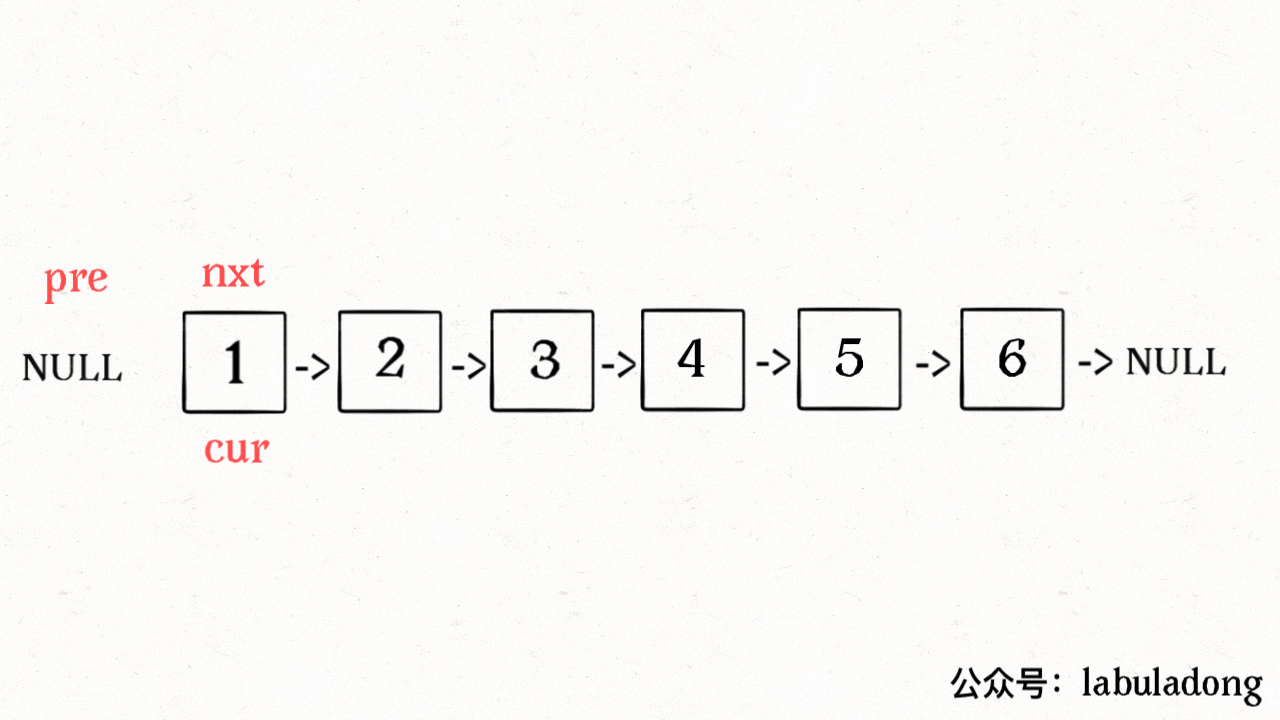「反转以 `a` 为头结点的链表」其实就是「反转 `a` 到 null 之间的结点」，那么如果让你「反转 `a``b` 之间的结点」，你会不会？

``````/** 反转区间 [a, b) 的元素，注意是左闭右开 */
ListNode reverse(ListNode a, ListNode b) {
ListNode pre, cur, nxt;
pre = null; cur = a; nxt = a;
// while 终止的条件改一下就行了
while (cur != b) {
nxt = cur.next;
cur.next = pre;
pre = cur;
cur = nxt;
}
// 返回反转后的头结点
return pre;
}
``````

``````ListNode reverseKGroup(ListNode head, int k) {
if (head == null) return null;
// 区间 [a, b) 包含 k 个待反转元素
ListNode a, b;
for (int i = 0; i < k; i++) {
// 不足 k 个，不需要反转，base case
if (b == null) return head;
b = b.next;
}
// 反转前 k 个元素
// 递归反转后续链表并连接起来
a.next = reverseKGroup(b, k);/**<extend up -90><div class="img-content"><img src="/algo/images/kgroup/6.jpg" class="myimage"/></div> */
}
``````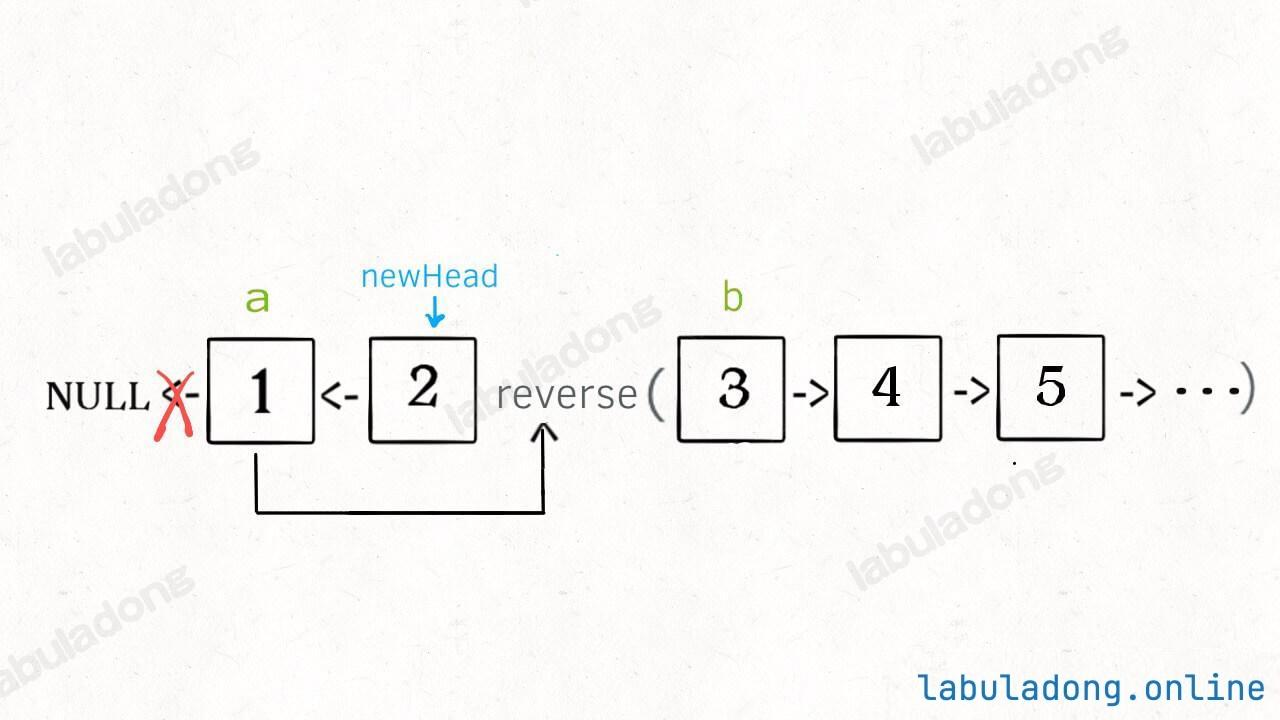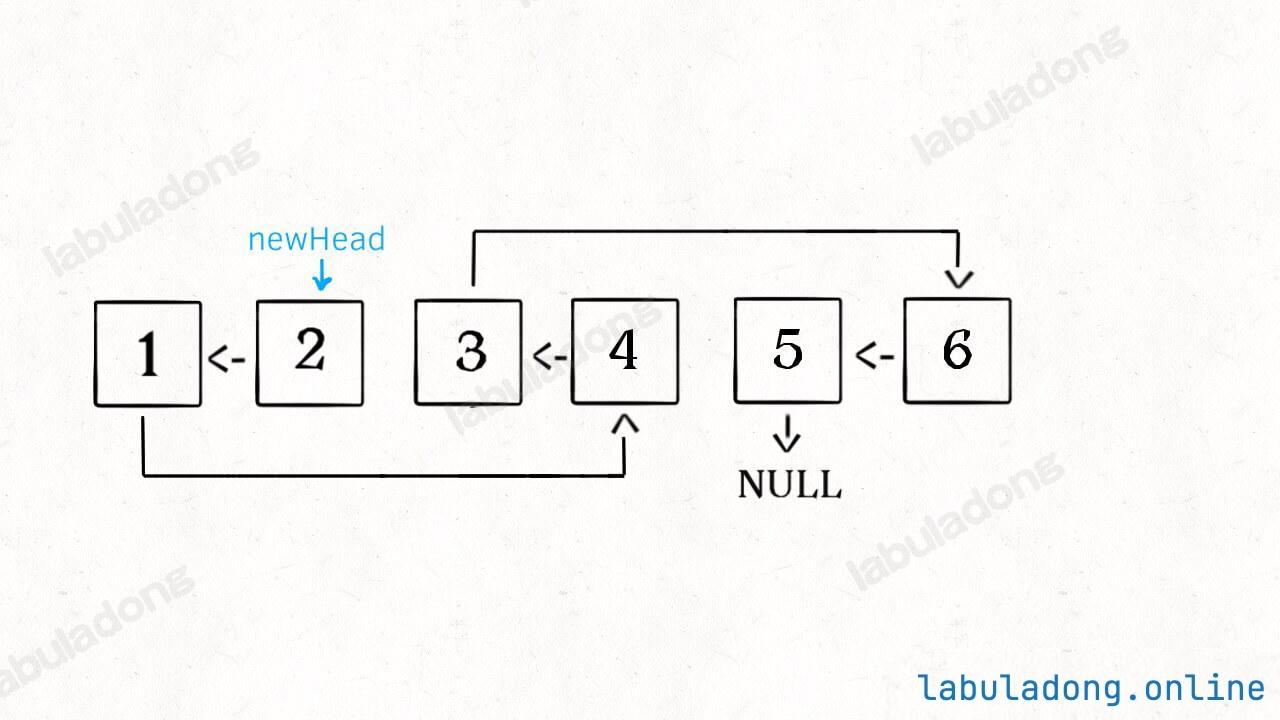### # 三、最后说两句